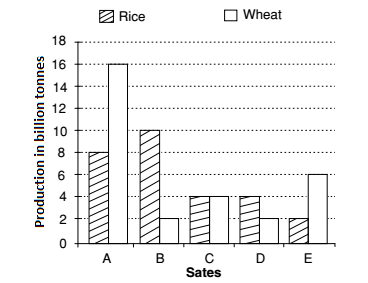## Bar graph

#### Data Interpretation

Direction: The bar graph provided below represents the production of rice and wheat in different states of a country in a certain year. Answer the question given below based on the bar graph.1. The average of production of rice in the mentioned states (in million tonnes) is:

1.  Average production of rice =Sum of the total production of rice in statestotal numbers of states

##### Correct Option: B

As per given bar graph , we can see that
The production of rice in states
A = 8 million tonnes
B = 10 million tonnes
C = 4 million tonnes
D = 4 million tonnes
E = 2 million tonnes

 Average production of rice =Sum of the total production of rice in statestotal numbers of states

 =8 + 10 + 4 + 4 + 2= 28 million tonnes 5 5

= 5.6 million tonnes

1. The difference between the production in rice and wheat is maximum in :

1. As per given graph we can check that which state have the Highest graph for Rice and Lowest graph for Wheat.
Required Difference = The production of rice - The production of wheat

##### Correct Option: D

As per given graph we can check that which state have the Highest graph for Rice and Lowest graph for Wheat.
From above given graph ,
The production of rice in state A = 16 billion tonnes
The production of wheat in state A = 8 billion tonnes
The production of rice in state B = 10 billion tonnes
The production of wheat in state B = 2 billion tonnes
∴ Required Difference = The production of rice - The production of wheat
State A ⇒ (16 − 8) = 8 billion tonnes
State B ⇒ (10 − 2) = 8 billion tonnes
Hence Answer will be states A and B both .

1. The total production of rice and wheat in all the mentioned states is minimum in the state.

1. From above given bar graph , we can see that
The graph for rice and wheat is lowest for state D.

##### Correct Option: C

From above given bar graph , we can see that
The graph for rice and wheat is lowest for state D.
Hence the total production of rice and wheat in all the mentioned states is minimum in the state D.
Hence Required answer will be state D.

1. The ratio of total production of rice in the mentioned states to that of wheat in those states, is :

1. Required ratio = Total production of Rice : Total production of Wheat

##### Correct Option: D

As per given bar graph , we can see that
Total production of rice in all state = (8 + 10 + 4 + 4 + 2) = 28 Lakhs tonnes
Total production of wheat in all state = (16 + 2 + 4 + 2 + 6) = 30 Lakhs tonnes
∴ Required ratio = Total production of Rice : Total production of Wheat
∴ Required ratio = 28 : 30 = 14 : 15

1. The state which is the largest producer of rice is :

1. From the given Bar graph, we can see that the Highest graph for Rice in all state will give the maximum production of Rice.

##### Correct Option: B

From the given Bar graph, we can see that the Highest graph for Rice in all state will give the maximum production of Rice. The production of rice is the Highest in state B.
Hence the state B is the largest producer of rice .
i.e. State B ⇒ 10 Lakhs tonnes